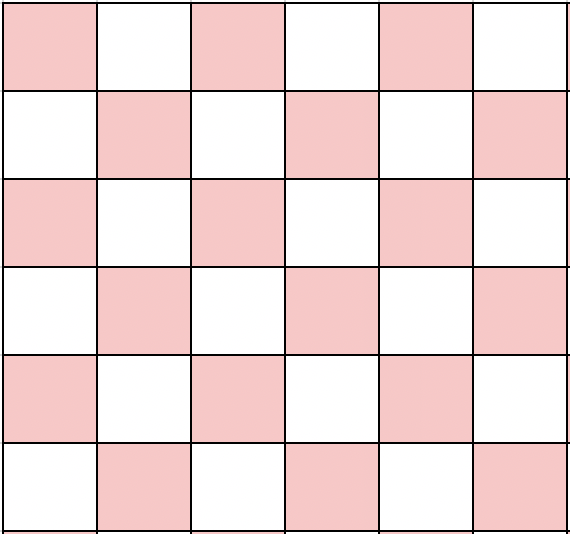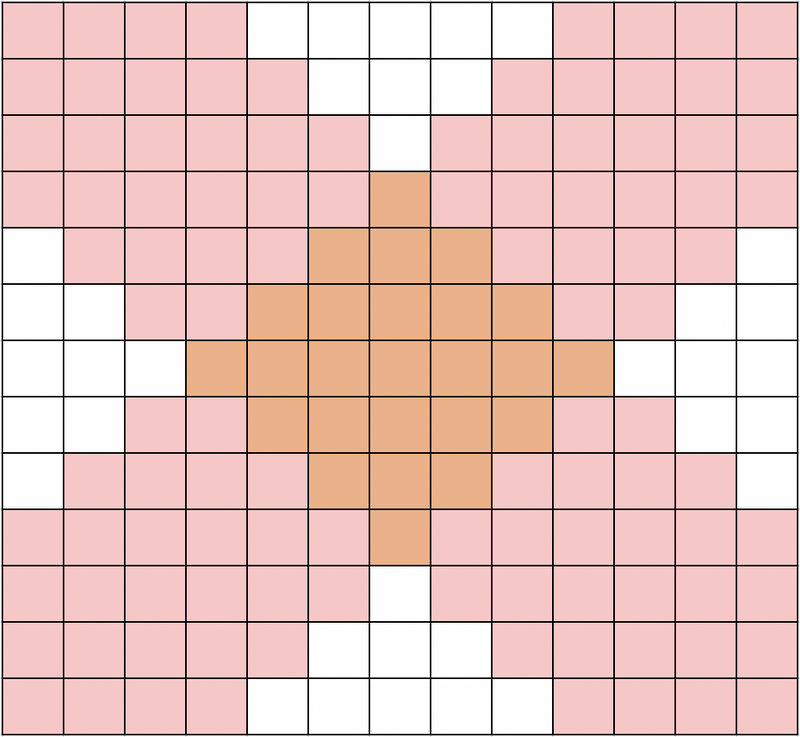Contest Duration: - (local time) (100 minutes) Back to Home

## C - Super Ryuma Editorial by evima

There are three conditions where it can move from square $$(a, b)$$ to square $$(c, d)$$:

• $$a + b = c + d$$
• $$a - b = c - d$$
• $$|a - c| + |b - d| \leq 3$$

Let us call them Move A, B and C, respectively.

First, we will focus on Moves A and B, which enable to move further.x By using Move A and Move B once each, it can travel to any square with the same parity (with the same color in the illustration below) in two moves. (It can be easily understood by rotating entire grid by $$45$$ degrees.)Therefore, with Move C combined, it can travel to any square in three moves.

Now that we know that the answer is at most three, all that left to solve this problem is to check if it can reach the goal in zero, one, or two moves. For zero moves, we can just check if the given squares are the same; for one move, we can check the three conditions written above. For two moves, we can split into several patterns:

• Move A + Move B:
• Whether the two squares have the same parity (the same color in the illustration above)
• $$(a + b + c + d) \bmod 2 = 0$$
• Move C + Move C:
• Whether the Manhattan distance is at most $$6$$
• $$|a - c| + |b - d| \leq 6$$
• Move A + Move C:
• See the illustration below
• $$|(a + b) - (c + d)| \leq 3$$
• Move B + Move C:
• See the illustration below
• $$|(a - b) - (c - d)| \leq 3$$By implementing them, you can obtain AC.

Sample Code (Python)

r1, c1 = map(int, input().split())
r2, c2 = map(int, input().split())
r = r2 - r1
c = c2 - c1
if (r, c) == (0, 0):
ans = 0
elif r == c or r == -c:
ans = 1
elif abs(r) + abs(c) <= 3:
ans = 1
elif (r ^ c ^ 1) & 1:
ans = 2
elif abs(r) + abs(c) <= 6:
ans = 2
elif abs(r + c) <= 3 or abs(r - c) <= 3:
ans = 2
else:
ans = 3
print(ans)


Sample Code (C++)

#include <bits/stdc++.h>
using namespace std;
int main(){
int r1, c1, r2, c2;
cin >> r1 >> c1 >> r2 >> c2;
int r = r2 - r1, c = c2 - c1;
int ans = 3;
if(!r && !c) ans = 0;
else if(r == c || r == -c || abs(r) + abs(c) <= 3) ans = 1;
else if((r ^ c ^ 1) & 1 || abs(r + c) <= 3 || abs(r - c) <= 3 || abs(r) + abs(c) <= 6) ans = 2;
cout << ans << endl;
}


posted:
last update: